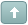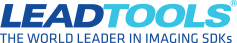Error

# best way to load/process several images?

#1 Posted : Wednesday, February 8, 2006 7:35:58 AM(UTC)

Hi, I'm new to both VB .net & LeadTools, and could use some advice.  I'm using LT Raster 14 (COM objects) in VB .net 2003.

I need to do the following for several JPG images (about 500 on average) that are each 2048x1360 pixels -

Open the image, perform some simple math, like calculate a ratio between the RGB bands (e.g. G +B / G-B) and write a new JPG that contains the results.  It all sounds really straight forward, but performance seems to be an issue...it takes about 8 minutes to do this per image using the following code (adapted for .net from a sample found on the lead support pages) -

imgInput.Load(arImageList(intLoop), 0, 0, 1) 'arImageList is a string array of file names

'copy the input for the classified image & fill with 0
imgClass.CreateBitmap(intCol, intRow, imgInput.BitmapBits)
imgClass.Fill(Convert.ToUInt32(RGB(Convert.ToInt32(0), Convert.ToInt32(0), Convert.ToInt32(0))))

intRow = imgInput.BitmapHeight
intCol = imgInput.BitmapWidth

For intX = 1 To intRow
For intY = 1 To intCol
color = Convert.ToInt64(imgInput.Pixel(intX, intY))
blue = (color) \ (2 ^ 16)
green = (color \ 256) And (&HFF)
red = color And (&HFF)
'**** IMAGE Band Ratio ALGORITHM HERE
'if ratio meets a threshold, output should be1 otherwise leave 0
imgClass.Pixel(intX, intY) = Convert.ToUInt32(RGB(1, 1, 1))

Next 'next column
Next 'next row

'*** save classified image

This works, but as I said is slow ( and the end users will probably have older & slower PCs than mine).  So...is there a better way to get to each pixel in an image?  Does Load() simply read pixel by pixel from the hard disk?  Would LoadArray() work better?  If so how do you determine the size for the vMem parameter? I've used both the InfoSizeDisk and InfoSizeMem properties but neither seem to work (I get cannot read past end of file errors).

Any and all suggestions would be appreciated.  Thanks!

Terry

 Try the latest version of LEADTOOLS for free for 60 days by downloading the evaluation: https://www.leadtools.com/downloadsWanna join the discussion? Login to your LEADTOOLS Support accountor Register a new forum account.#2 Posted : Sunday, February 12, 2006 4:23:14 AM(UTC)
Terry,
You could use LEADTOOLS image processing functions.
For example, instead of going pixel-by-pixel, use the following functions (all in Main Control):

1. Use the ColorSeparate method to get the R, G and B planes into 3 separate 8-bit images.

2. Use the Combine method on the resulting images with needed flags, such as:
CB_OP_SUBSRC    Subtract the source from the destination, allowing a minimum of 0.
CB_OP_SUBDST    Subtract the destination from the source, allowing a minimum of 0.

CB_OP_MUL    Multiply the byte values, allowing a
maximum of 255. The result is calculated as follows: result = (source * dest) / 255.

CB_OP_DIVSRC    Divide the destination by the source. If
source > 0, then the result is calculated as follows: result = min(
(dest / source) * 255, 255 ). Otherwise, if source = 0, then result = 255.

CB_OP_DIVDST    Divide the source by the destination. If
dest > 0, then the result is calculated as follows: result = min( (source / dest) * 255, 255 ). Otherwise, if dest = 0, then result = 255.

3. To perform the final threshold calculation on the resulting image, use the IntensityDetect method

This way, you will be calling one LEADTOOLS method per mathematical step that does all pixels in one shot.
Amin Dodin

Senior Support Engineer